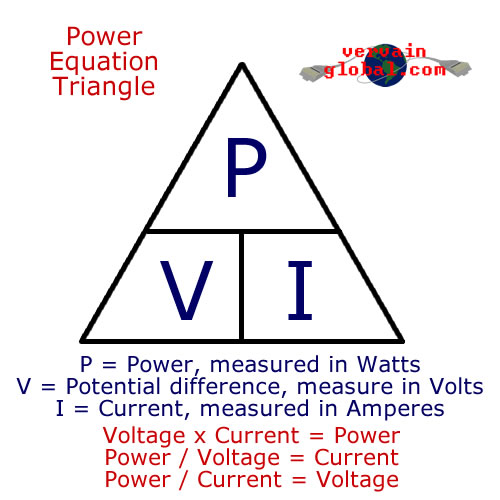# Basic electrical principles explained

This page will teach you the fundamental basics of how electricity works. It will help you to understand the relationship and differences between Voltage, Current and Power.### Measuring electricity

You'll almost certainly have heard of Volts, Amps and Watts. These units of measurement are all ways of monitoring electricity in some way. Despite that, they all measure something different. Knowing the importance and difference of these units will give you a whole new understanding of electricity, and how some of your electrical appliances work.

### Units of measure

First up is the Volt. Everyone's heard of this one. The name was coined by the physicist Alessandro Volta in 1792, shortly before he invented the first battery. A "volt" is infact a measurement of potential difference. That is, it measures the difference in electrical charge between two conductors. If you were to visualise electricity as a liquid, potential difference is like pressure. The pressure of 2 volts would be twice that of the pressure of 1 volt. The symbol for voltage is the capital "V"

Next is Current. Current is measured in amperes, or amps. This one was named after the French mathematician, Andre-Marie Ampere. If voltage is like pressure, current is like flow. As electricity flows faster through a cable, current rises. The symbol for current is the capital letter "I".

Then we have Power. Power is a measurement of how much "work" a source of energy is performing. When a source of energy changes form, for example electrical energy being turned into heat and light energy by a light bulb, we can measure how fast that energy is changing form. Power can be measured in several different units, including the Joule and the Horsepower. In electricity, the Watt is by far the most commonly used. This one was named after the Scottish engineer, James Watt. The symbol "P" is often used to represent power, but you will sometimes see the letter "W".